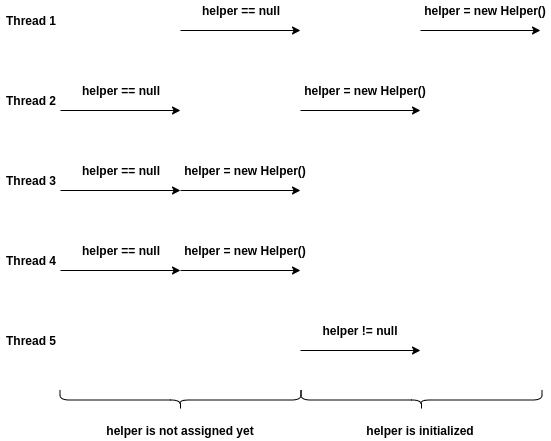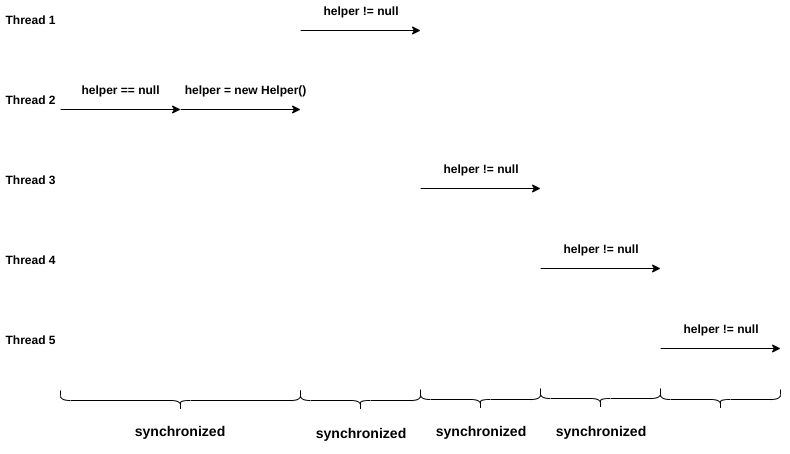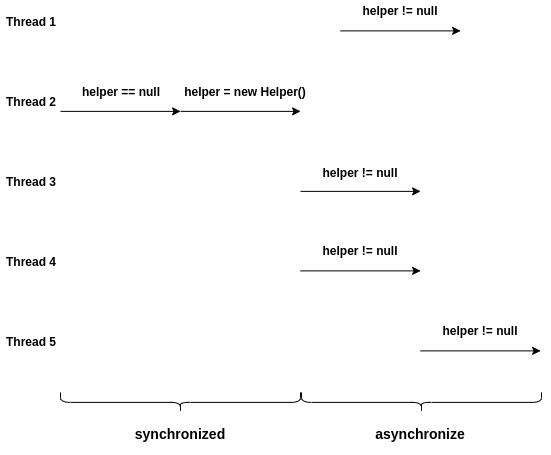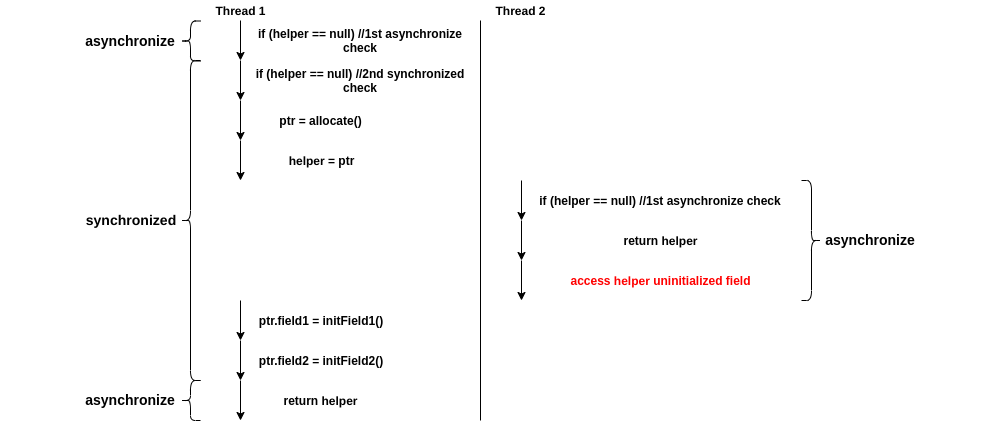# Double-Checked Locking is Broken

Double-checked locking is a software design pattern for reducing the overhead of acquiring a lock. The program checks locking criteria first, and acquires the lock only if the check indicates that locking is required.

Lazy initialization is a commonly used tactic for delaying the object initialization until the first time it is accessed. In multi-threaded environments, initialization is usually not thread safe, so locking is required to protect the critical section. Since only the first access requires locking, double-checked locking is used to avoid locking overhead of subsequent accesses. However, on many languages and hardware, the design can be unsafe.

If we were writing single-threaded code, we could write a lazy initialization like this:

```class Foo {
private Helper helper = null;
public Helper getHelper() {
if (helper == null)
helper = new Helper();
return helper;
}
}
```

This code works for a single thread, but if the code is run in multi-threaded environments, two or more threads could find that `helper` is `null` at the same time, and create multiple copies of `Helper` object. This can even cause a memory leak in some languages, such as C++.As shown in the graph above, when threads either run concurrently on single processor (e.g., thread 1 and thread 2), or run in parallel on different processors simultaneously (e.g. thread 3 and thread 4), they can create multiple copies of `helper`.

### Always-synchronized solution is slow

To fix this issue, we can simply add a lock to this critical section as follows, so that only one thread can enter this critical section at a time.

```class Foo {
private Helper helper = null;
public Helper getHelper() {
synchronized(this) {
if (helper == null)
helper = new Helper();
return helper;
}
}
}
```However, we only need this section of code to be synchronized for the first thread access. After the object is created, acquiring and releasing lock is unnecessary, and they can have a huge performance impact.

What we want is something like this:Only the first thread will enter the synchronized section and create the object. Once the `helper` is initialized, all subsequent accesses can run in parallel without synchronization.

Intuitively, we can come up with the following steps to do this job:

1. Check if the object is initialized without locking. If it is, then return the object immediately.
2. Acquire the lock and check again if the object is initialized. If another thread has previously grabbed the lock, the current thread can see the object is created, and return the object.
3. Otherwise, the current thread will create the object and return.

With the guidelines above, we will get the following code:

```class Foo {
private Helper helper = null;
public Helper getHelper() {
if (helper == null) {              // first check
synchronized(this) {
if (helper == null)         // second check
helper = new Helper();
}
}
return helper;
}
}
```

This tactic is called double-checked locking.

### Double-checked locking is broken

However, this code is not guaranteed to work.

`helper = new Helper()` is not an atomic operation, it consists of multiple instructions allocating space, initializing fields of the object, and assigning address to `helper`.

In order to show what is really happening there, we expand `helper = new Helper()` with some pseudocode and inline the object initialization code.

```class Foo {
private Helper helper = null;
public Helper getHelper() {
if (helper == null) {
synchronized(this) {
if (helper == null) {
ptr = allocate();
ptr.field1 = initField1();
ptr.field2 = initField2();
helper = ptr;
}
}
}
return helper;
}
}
```

In order to improve overall performance, some compilers, memory systems, or processors may reorder the instructions, like moving `helper = ptr` before initializing fields of the object.

```class Foo {
private Helper helper = null;
public Helper getHelper() {
if (helper == null) {
synchronized(this) {
if (helper == null) {
ptr = allocate();
helper = ptr;
ptr.field1 = initField1();
ptr.field2 = initField2();
}
}
}
return helper;
}
}
```

This reordering is legal because there is no data dependency between `helper = ptr` and the instructions for initializing fields. However, this reordering, in some certain execution order, could result in other threads seeing a non-null value of `helper` but accessing uninitialized fields of the object.### Another fix is also broken

A memory barrier is a type of instruction that can make the compiler and processor enforce the ordering, so that the instructions on one side of the memory barrier will not be reordered to the other side of the barrier.

In order to enforce that object initialization `new Helper()` to execute before assigning to `helper`, some people came up with another fix with a `synchronized` to enforce ordering, since `synchronized` is an implicit memory barrier that enforces the instructions inside the synchronized section to be executed before exiting the section (i.e., releasing the lock).

```class Foo {
private Helper helper = null;
public Helper getHelper() {
if (helper == null) {
Helper h;
synchronized(this) {
h = helper;
if (h == null)
synchronized(this) {
h = new Helper();
}
helper = h;
}
}
return helper;
}
}
```

The purpose of second `synchronized` is only to create memory barrier, since mutual exclusion is already enforced by the first `synchronized`.

The intuition is that the lock releasing would act as a memory barrier, so that `helper=h` will not be executed until the initialization in the synchronized section is done.

Unfortunately, the lock releasing is a one-way memory barrier on many processors. It only enforces the instructions in the synchronized section to be executed before lock is released. The instruction `helper=h` behind the memory barrier could still be moved into synchronized section and executed before the object initialization is done.

The expanded and reordered pseudocode should look like the following, which will result in the same problem as the original version of double-checked locking.

```class Foo {
private Helper helper = null;
public Helper getHelper() {
if (helper == null) {
Helper h;
synchronized(this) {
h = helper;
if (h == null)
synchronized(this) {
ptr = allocate();
h = ptr;
helper = h;
ptr.field1 = initField1();
ptr.field2 = initField2();
}
}
}
return helper;
}
}
```

## Working Solutions

### Explicit Memory Barrier

The previous fix with two synchronized sections does not work because releasing a lock is an implicit "one-way" memory barrier. It is possible to make the double-checked locking actually work with an explicit memory barrier. For example, Preshing has provided an implementation of double-checked locking with `std::atomic` and `std::atomic_thread_fence` in C++ 11.

```class Foo {
private:
std::atomic <Foo*> helper;
public:
Foo* get_helper() {
if (h == nullptr) {
std::lock_guard<std::mutex> lock(m_init);
if (h == nullptr) {
h = new Helper;
helper.store(h, std::memory_order_relaxed);
}
}
return h;
}
};
```
• `std::atomic_thread_fence(std::memory_order_acquire)` guarantees that read/write operations after a memory barrier cannot be reordered with read operations before the memory barrier.
• `std::atomic_thread_fence(std::memory_order_release)` guarantees that read/write operations before a memory barrier cannot be reordered with write operations after the memory barrier.

The memory barriers guarantee that

1. `h` loads the value from `helper` before starting object initialization.
2. object initialization finishes before storing the value to `helper`.

### Atomic Operation

An atomic operation will either happen completely, or it does not happen at all. This is no intermediate state, so that the side effects of an atomic operation will not be visible until the operation is complete.

In previous analysis, we have seen that `h = new Helper()` can be interleaved because it is not an atomic operations. If this operation is atomic, the double-checked locking will work.

#### Volatile

Since JDK 5, we can make reads and writes for any variable atomic by declaring it as a volatile variable. Every read of a volatile will invalidate cached value and load it from main memory. Every write of a volatile will update value in cache and then flush out the cached value to main memory.

The "volatile" in Java also provides ordering guarantees, which are the same guarantees `atomic_thread_fence` in C++ provides:

• The read/write operations of other variables after a read from a volatile variable cannot be reordered before the read from the volatile variable.
• The read/write operations of other variables before a write to a volatile variable cannot be reordered after the write to the volatile variable.

With this new feature, the double-checked locking issue is resolved by simply declaring `helper` as a volatile variable.

```class Foo {
private volatile Helper helper = null;
public Helper getHelper() {
if (helper == null) {
synchronized(this) {
if (helper == null)
helper = new Helper();
}
}
return helper;
}
}
```

However, since all read and write operations of a volatile variable triggers cache coherence protocol and accesses main memory, it can be very slow. An improvement can be done with a local variable, to reduce number of times accessing volatile variable.

```class Foo {
private volatile Helper helper = null;
public Helper getHelper() {
Helper h = helper;
if (h == null) {
synchronized(this) {
h = helper;
if (h == null) {
h = new Helper();
helper = h;
}
}
}
return h;
}
}
```

In cases that the `helper` is already initialized, this optimization can reduce one volatile read by returning the local variable.

#### 32-bit Primitive Variables

Pugh et al. claimed that the double-checked locking can work safely for 32-bit primitives.

```class Foo {
private int magicNumber = 0;
public int getMagicNumber() {
if (magicNumber == 0) {
synchronized(this) {
if (magicNumber == 0)
magicNumber = GetMagicNumber();
}
}
return helper;
}
}
```

However, this specific case highly depends on the Java memory model. The C/C++ equivalent of the above code is not safe. The Java documentation specifies that read and write operations of most primitive variables (except `long` and `double` since they are 64-bit) are atomic. But it is still not completely clear why it is safe and how it is different from volatile primitive variables.

### Static Singleton

If the `helper` is static, i.e., all the instances of class `Foo` share the same instance of `helper`, defining the `helper` in a static field of a separate class will solve the problem.

```class Foo {
private static class HelperSingleton {
public static final Helper helper = new Helper();
}

public Helper getHelper() {
return HelperSingleton.helper;
}
}
```

This is known as initialization-on-demand holder idiom, which is considered as a safe and efficient concurrent lazy initialization for all Java versions.

As the Java documentation specifies, a lock is used to ensure synchronized access to object initialization status (uninitialized/initializing/initialized/erroneous state). However, if all subsequent references to the object requires lock synchronization, it is just equivalent to the "always-synchronized" solution above. Unfortunately, it is still unclear how Java provides an efficient unsynchronized access to initialized objects.

Alexander Terekhov provided an implementation of double-checked locking using thread local variables.

`ThreadLocal` is a variable where each thread will have its own copy of the thread local variable. Each thread can only access and modify its own copy of a thread local variable independently of other threads.

A thread local can be used to maintain the state of "whether the state has gone through the synchronized initialization or not". If a thread has gone through the synchronized initialization once, it can be confident that that object is already initialized.

Inside the synchronized initialization section, only the first thread will find the object is `null` and initialize the object. All threads will then change their per-thread state at the first synchronized access, so that they will not enter the synchronized section again.

```class Foo {
private Helper helper = null;
public Helper getHelper() {
synchronized {
if (helper == null)
helper = new Helper();
}
}
return helper;
}
}
```

Admittedly, this solution is slightly more costly compared with the "ideal" design: instead of having only the first thread enter the synchronized section and initialize the object, each thread is required to enter the synchronized section exactly once and change its own per-thread state (i.e., the thread local variable) to prevent future access of the synchronized section. However, the performance in the long run is still acceptable.

## Conclusion

The article discusses the problem of double-checked locking for lazy initialization in multi-threaded environments. It analyzes why some intuitive solutions do not work, and also analyzed some working solutions.

Writing multi-threaded program is hard. Writing correct and safe multi-threaded program is even harder. When analyzing the correctness of multi-threaded programs, it requires the considerations of multiple components, including compilers, systems, and processors. On the other hand, when designing compilers, systems, or processors, one also needs to take into consideration commonly used design patterns.

## Acknowledgement

I have referred following documents for code examples and explanations. Code structures and variable names are modified for consistency in this post.